# Altitude Of A Triangle

## What is Altitude Of A Triangle?

Definition: Altitude of a triangle is the perpendicular drawn from the vertex of the triangle to the opposite side. Also, known as the height of the triangle, the altitude makes a right angle triangle with the base. Below is an image which shows a triangle’s altitude.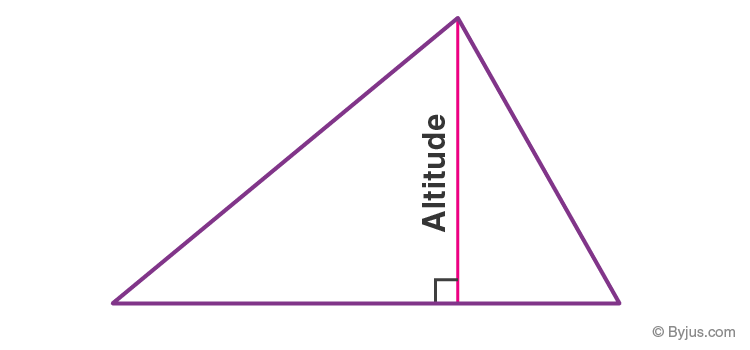### What is the Use of Altitude of a Triangle?

The main use of the altitude is that it is used for area calculation of the triangle, i.e. area of a triangle is (½ base × height). Now, using the area of a triangle and its height, the base can be easily calculated as Base = [(2 × Area)/Height]

## Altitudes of Different Triangles

About altitude, different triangles have different types of altitude. Below is an overview of different types of altitudes in different triangles.

### Altitude of an Obtuse Triangle

For an obtuse-angled triangle, the altitude is outside the triangle. For such triangles, the base is extended, and then a perpendicular is drawn from the opposite vertex to the base. For an obtuse triangle, the altitude is shown in the triangle below.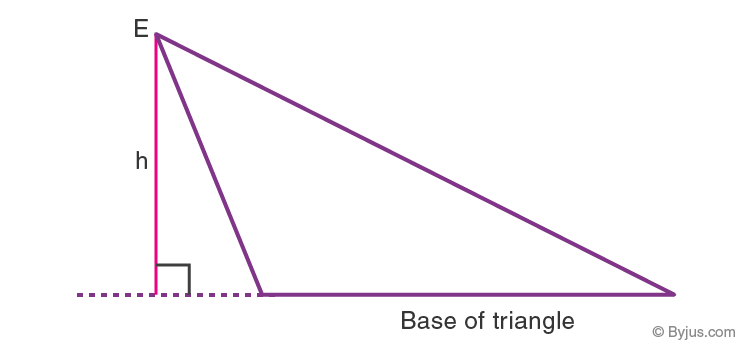### Altitude of an Equilateral Triangle

The altitude or height of an equilateral triangle is the line segment from a vertex that is perpendicular to the opposite side. It is interesting to note that the altitude of an equilateral triangle bisects its base and the opposite angle. The image below shows an equilateral triangle ABC where “BD” is the height (h), AB = BC = AC, ∠ABD = ∠CBD, and AD = CD.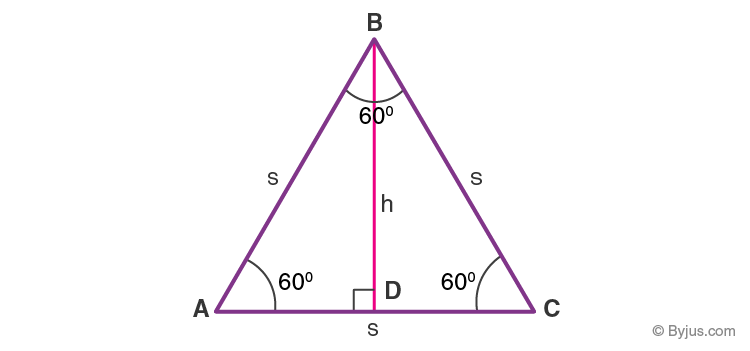### Altitude of a Right Triangle

The altitude of a right-angled triangle divides the existing triangle into two similar triangles. According to right triangle altitude theorem, the altitude on the hypotenuse is equal to the geometric mean of line segments formed by altitude on hypotenuse.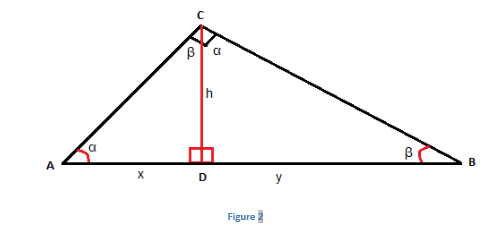Altitude of a Right-Angled Triangle

### Altitude of an Isosceles Triangle

The isosceles triangle altitude bisects the angle of the vertex and bisects the base. It should be noted that an isosceles triangle is a triangle with two congruent sides and so, the altitude bisects the base and vertex.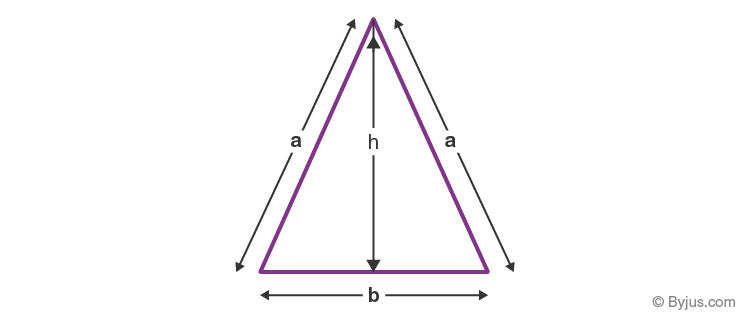## Altitudes of Triangles Formulas

Triangle Type Altitude Formula
Equilateral Triangle h = (½) × √3 × s
Isosceles Triangle h =√(a2−b2⁄2)
Right Triangle h =√(xy)

A brief explanation of finding the height of these triangles are explained below.

### Altitude of an Equilateral Triangle Formula

For an equilateral triangle, all angles are equal to 60°.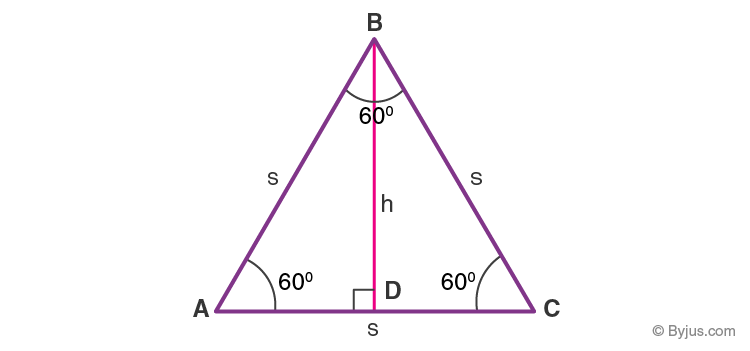sin 60° = h/AB
We know, AB = BC = AC = s (since all sides are equal)
∴ sin 60° = h/s
√3/2 = h/s
h = (√3/2)s

⇒ Altitude of an equilateral triangle = h = √(3⁄2) × s

Click now to check all equilateral triangle formulas here.

### Altitude of a Right Triangle Formula

To calculate the area of a right triangle, the right triangle altitude theorem is used.

⇒ Altitude of a right triangle =  h = √xy

Keep visiting BYJU’S to learn various Maths topics in an interesting and effective way. Also, register now and download BYJU’S – The Learning App to get engaging video lessons and personalised learning journeys.